# Test: Basic Concepts - 1

## 10 Questions MCQ Test Network Theory (Electric Circuits) | Test: Basic Concepts - 1

Description
Attempt Test: Basic Concepts - 1 | 10 questions in 30 minutes | Mock test for Electrical Engineering (EE) preparation | Free important questions MCQ to study Network Theory (Electric Circuits) for Electrical Engineering (EE) Exam | Download free PDF with solutions
QUESTION: 1

### A solid copper sphere, 10 cm in diameter is deprived of 1020 electrons by a charging scheme. The charge on the sphere is

Solution:

n = 1020 ,  Q =  ne = 1020 e (e = 1.6 x 10-19 )
= 16.02 C
The charge on the sphere is 16.02 coulomb. It will be positive.

QUESTION: 2

### A lightning bolt carrying 15,000 A lasts for 100 μs. If the lightning strikes an airplane flying at 2 km, the charge deposited on the plane is

Solution: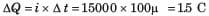QUESTION: 3

### If 120 C of charge passes through an electric conductor in 60 sec, the current in the conductor is

Solution: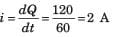QUESTION: 4

The energy required to move 120 coulomb through 3V is

Solution:

W = Qv = 360J

QUESTION: 5

i = ?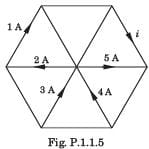Solution: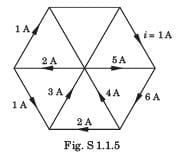QUESTION: 6

In the circuit of fig P1.1.6 a charge of 600 C is delivered to the 100 V source in a 1 minute. The value of v1 must be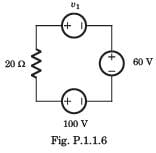Solution:

In order for 600 C charge to be delivered to the 100 V source, the current must be anticlockwise.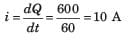Applying KVL we get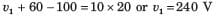QUESTION: 7

In the circuit of the fig P1.1.7, the value of the voltage source E is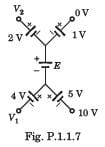Solution:

Going from 10 V to 0 V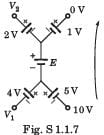10 + 5 + E + 1 + 0 = 0 or E =-16 V

QUESTION: 8

Consider the circuit graph shown in fig. P1.1.8. Each branch of circuit graph represent a circuit element. The value of voltage v1 is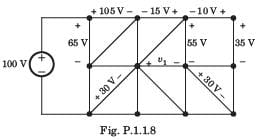Solution: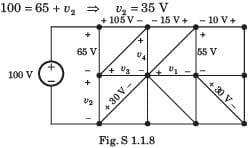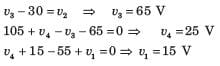QUESTION: 9

For the circuit shown in fig P.1.1.9 the value of voltage vo is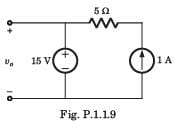Solution:

Voltage is constant because of 15 V source.

QUESTION: 10

R1 = ?​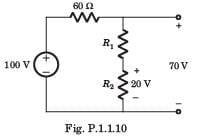Solution:

Voltage across 60 Ω resistor = 30 V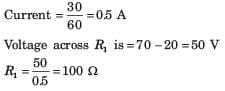Use Code STAYHOME200 and get INR 200 additional OFF Use Coupon Code